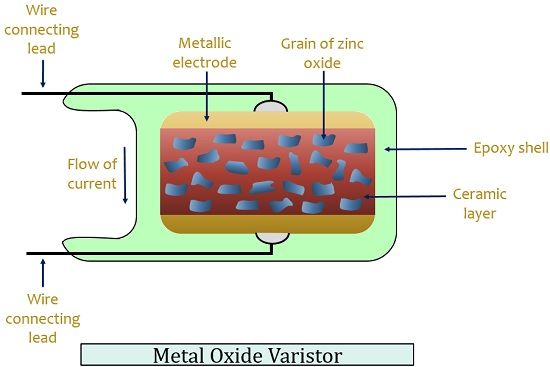# Varistor

Definition: A varistor is a 2 terminal semiconductor device that protects the electrical and electronic devices from overvoltage transients. Its resistance is dependent on the applied input voltage.

The word varistor is formed by the combining variable and resistor. It is also known as voltage-dependent resistor, VDR whose resistance changes automatically with the corresponding change in the voltage across it.

It is always placed in shunt with the device being protected. This is basically done to protect the circuit from voltage surges.

The figure below shows the symbolic representation of a varistor:These are basically used to protect the circuit from high voltage fluctuations.

## Construction of Varistor

Varistors are formed when crystals of silicon carbide or metal oxides are pressed into a ceramic material.

Then sintering of the material is done at high temperature after it gets dried. The electrical characteristics of the device are dependent on temperature provided and atmospheric conditions.

In order to have good electrical contacts, the contacts of the material are metallized with silver or copper. Then leads are soldered to the contacts and varistors are furnished and coded.

The figure below shows a disc type varistor:Nowadays these are the most common voltage clampers and can be used for a wide range of voltage. It is a non-linear device and absorbs the destructive energy and dissipates it in the form of heat in order to prevent system damage.

Usually, zinc oxide is used in its manufacturing thus is also known as metal oxide varistor.

The figure below shows the structure of metal oxide varistor:Here the varistor is formed 90% with zinc oxide and the rest is filler material that forms the junction. A standard silicon carbide is different from the metal oxide varistor in the way that a MOV exhibits less leakage current and its operational speed is faster.

## Operation and Characteristic of Varistor

Before proceeding towards its operation, let us first understand the relationship between voltage and resistance of varistors.

The figure below shows resistance versus voltage curve for a varistor:Varistors exhibit unusual behaviour in case of resistance. Here as we can see that when the voltage is low then the resistance across it is high. But the resistance drops rapidly with the increase in voltage above rated voltage.

Let us now have a look at the detailed operation of varistor:

When a certain low voltage is applied across the device then it offers high resistance due to which a very low current flows through it. As the voltage increases and reaches clamping voltage i.e., the rated voltage, the current now increases.

At this particular time, a change in the operation of the varistors is noticed. So, after this voltage, the device which is till now behaving as an insulator now starts behaving as a conductor. So, after rated voltage the resistance offered by it will become very low, allowing very high current to pass through it.

Thus, it is said that voltage shows non-linear characteristic with current.

The figure below shows the current versus voltage characteristic of a varistor:Here, as we can see that until the clamping voltage is reached, the device remains in the non-conducting state. Thus we can see a linear relationship between voltage and current. At this time a leakage current of very low value flows through it. Due to the high resistance offered by it.

However, after this particular voltage level, conducting state is achieved by the varistors. Thus we can see that the resistance has become very low and large current is flowing through it even after the voltage is limited after the rated voltage.